﻿ Time Series Forecasting (1): Initial analysis – Data Science Austria

# Time Series Forecasting (1): Initial analysis

I will be using Pandas through the entire notebook to handle the data and matplotlib for the visualization.

```import numpy as npimport pandas as pdimport matplotlib.pyplot as plt
```

Let’s start by loading the data a having a quick look at its shape and first values. Since I already know this is a time series I’m going to set the datetime column as the index.

```temp = pd.read_csv('../input/temperature.csv', parse_dates=['datetime'])temp = temp.set_index('datetime')print('Dataset shape: {}'.format(temp.shape))temp.head()
```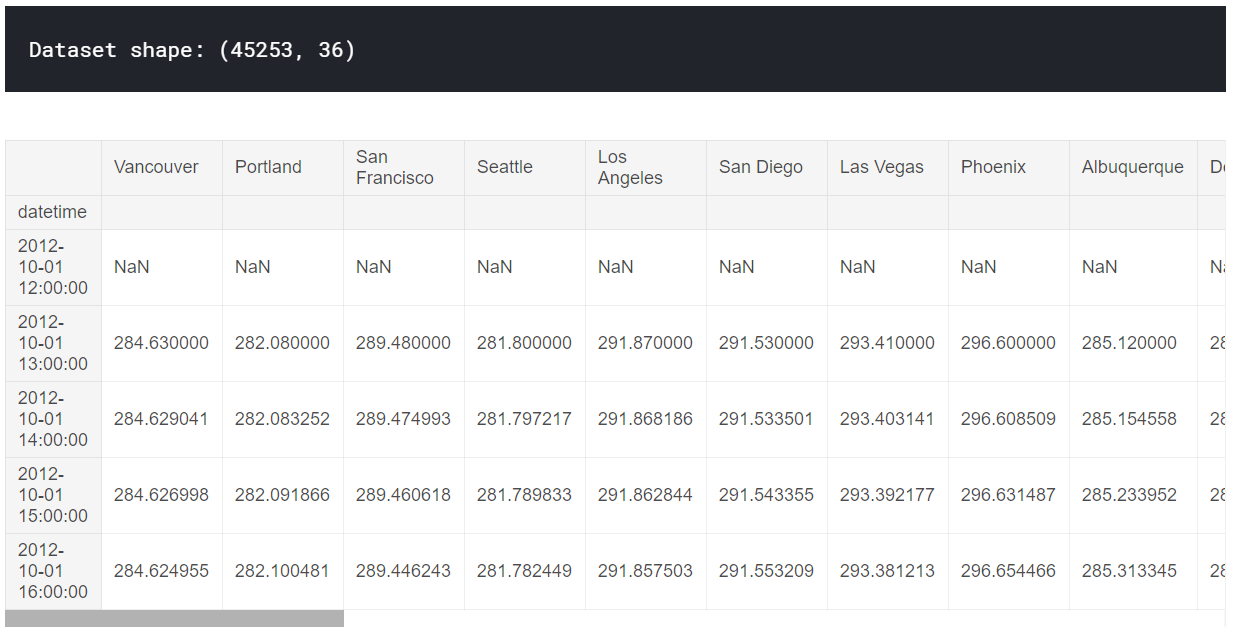This dataset is composed by 36 columns, corresponding to 36 different cities, and 45253 rows, giving the value of the temperature every hour from late 2012 to late 2017.

As I said, one of the cities I’m going to use is the one with the highest variation in yearly temperature. Let’s find out which one.

```all_std = temp.std(axis=0)max_std = all_std.max()city_max_std = temp.columns[all_std==max_std]
```
```print('City with highest temperature variation: {} ({} degrees)'.format(city_max_std,round(max_std,2)))
```Let’s subset the data now so it only contains values for San Francisco and Minneapolies and see some statistics.

```data = temp[['San Francisco','Minneapolis']]data.describe()
```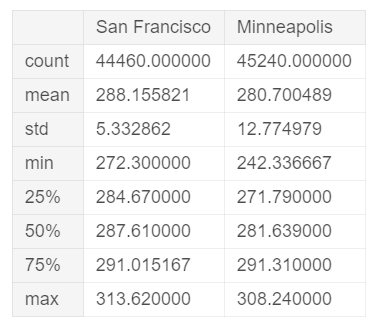First thing I see is that there are missing data in both columns, I’ll deal with that soon. Also, this temperature values are obviously not in a normal scale for day-to-day temperature. Since I live in Europe I’m going to transform the data from Kelvin to Celsius (sorry if you use Farenheit, but International Systemm baby).

```data = data-273.15data.describe()
```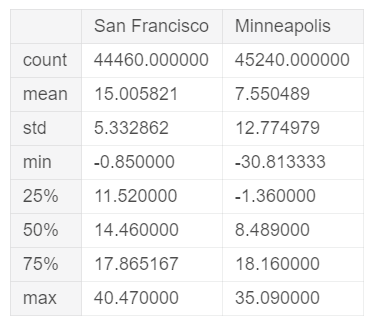### Visualizing the data

Let’s see what the temperature for these two cities looked like along the years.

```_=data.plot(figsize=(15,5),subplots=False,title='Temperature',alpha=0.7)_=plt.xlabel('Date')_=plt.ylabel('Temperature')
```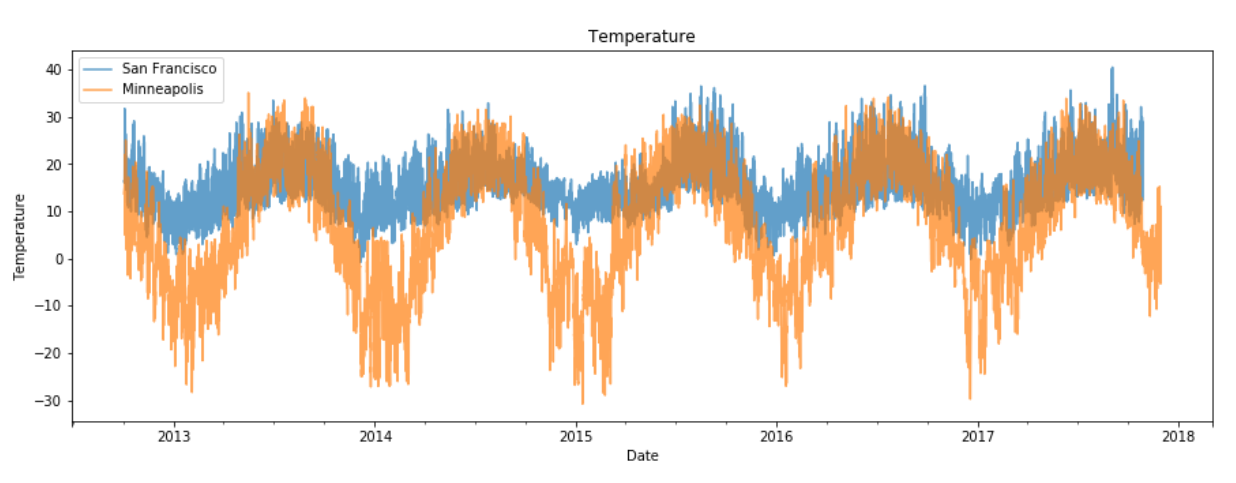Not bad, the weather seems quite cold in Minneapolis, maybe I wouldn’t go there during the winter.

Also, there is a clear seasonality in the data with a period of a year. There is also more variation, I’ll check later if this variation has something to do with some daily seasonality or it’s more random.

Something I can see in this figure is that we have less data for San Francisco. The blue line doesn’t get that close to 2018.

### Cleaning the data

As we saw, there are clearly missing values, something that caughts my attention in the figure may be a reason for this. As I mentioned, the data for San Francisco finishes earlier, to work with both series at the same time I’m going to lose the final values in the Minneapolis series.

To do so I’m going to keep all the non-missing values of San Francisco and see the maximum date they reach. Then, we’ll cut all data with date larger than that.

```SF_non_missing = data['San Francisco'].dropna()max_date = SF_non_missing.index.max()data = data[data.index <= max_date]
```

Let’s see if we still have missing values.

```print(data.isna().sum())
```Ok, we still have to deal with the problem of missing data, but there is something I want to deal with first. My intention here is to study the yearly behaviour of the data, I’m not interested in the daily variation, so I’m going to resample the data into a daily frequency by taking the average, minimum and maximum of all the temperatures through that day.

```data_mean = data.resample('D').mean()data_min = data.resample('D').min()data_max = data.resample('D').max()print('Resample shape: {}'.format(data_mean.shape))data_mean.describe()
```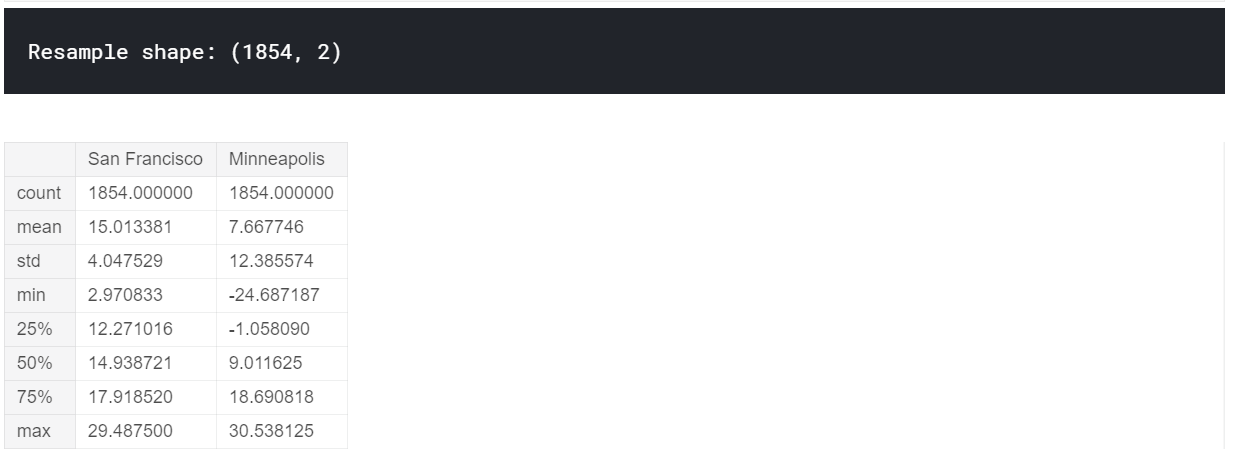This could also have solved our problem with missing data, if there was more any row with non-missing data everyday. Let’s check.

```print('Missing data now?')print(data_mean.isna().sum())
```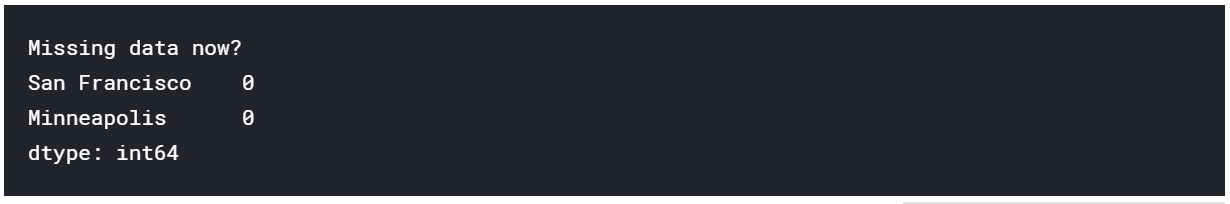No missing data now! This means that we have at least one value per day.

If this were not the case I would have used the values from the previus day. I like this solution for time series more than just dropping the row (is better to have the same temporal separation between all rows) or just using the mean value (since this would mess with the shape of the curve).

Let’s check how our data looks after resampling.

```_=data_mean.plot(figsize=(15,5),subplots=False,title='Temperature',alpha=0.7)_=plt.fill_between(x=data_mean.index,y1=data_min['San Francisco'].values,y2=data_max['San Francisco'].values,alpha=0.3)_=plt.fill_between(x=data_mean.index,y1=data_min['Minneapolis'].values,y2=data_max['Minneapolis'].values,color='orange',alpha=0.3)_=plt.xlabel('Date')_=plt.ylabel('Temperature')
```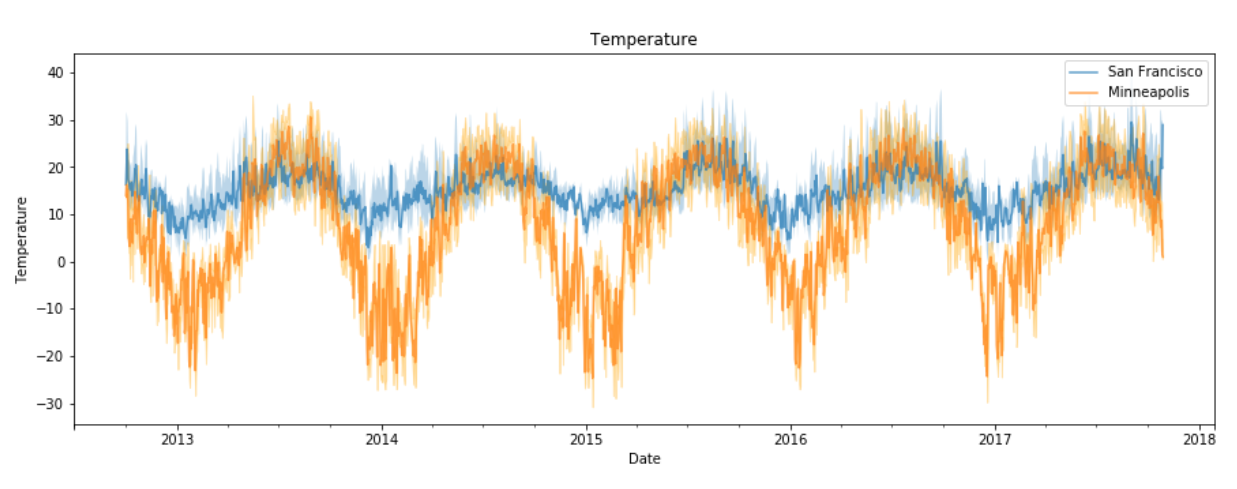The shadow around the curve shows the min-max values during that day, while the main line shows the mean value.

Now both curves end at the same point, and we have less daily variation.

### Outliers

Some times weird things happen and we end up with values that can mess up an entire model. One sensor can fail, for example, and measure a temperature of -10º in summer, which definitely is not normal. Other times you can see 10º in the summer, that’s low, but maybe not an error, sometimes it can get cold, you can’t remove this type of value, because there can be some reason to it. We have to be carefull how we treat outliers, we shouldn’t remove it unless we know it’s an error or is a one-time-thing that shouldn’t affect our model.

It’s not always easy to identify which points are outliers, and what to do with them, but a good place to start is to inspect the data to see if there are points with extremely high or low values. One good way to see this visually is to use histograms.

```_=plt.hist(data_mean['San Francisco'], alpha=0.5, label='San Francisco')_=plt.hist(data_mean['Minneapolis'], alpha=0.5, label='Minneapolis')_=plt.legend()
```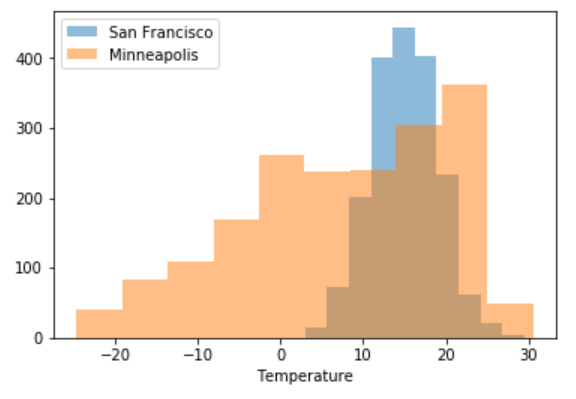Let’s look into each of the cities individually:

• Values for San Francisco seem to follow a gaussian distribution with a small standard deviation, we can’t see any outliers in this data.
• For Minneapolis the curve is less perfect, with a high skewness to the right side (negative skewness). We cannot say that any of this points are outliers though, since there is quite a few of them, also, in the visual representation of the temperatures we could see that really low values are reached every year.

I don’t see any outliers and don’t think I should remove any points.

### Looking for stationarity and autocorrelation

Ths gaussian-shaped histagram we plotted earlier is a first clue the time series can be stationary.

Another clue is to compute some statistics on the time series in different time ranges and looking for a variation.

```cut = data_mean.index[int(0.5*len(data_mean))]print('Mean before {}:'.format(cut))print(data_mean.loc[:cut].mean())print('')print('Mean after {}:'.format(cut))print(data_mean.loc[cut:].mean())print('')print('---------------------------')print('')print('Std before {}:'.format(cut))print(data_mean.loc[:cut].std())print('')print('Std after {}:'.format(cut))print(data_mean.loc[cut:].std())
```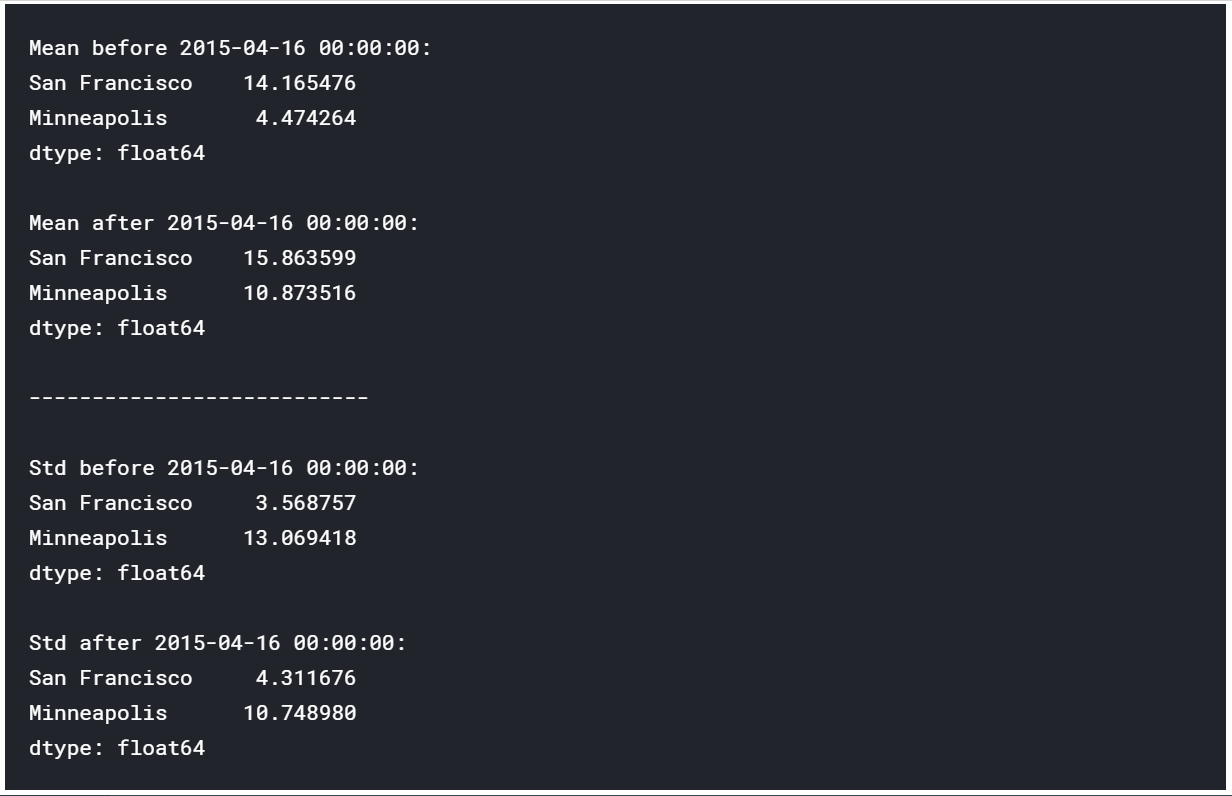We can see that the values are pretty close for San Francisco, but further away for Minneapolis, they are still close enough for the time series to be stationary since we need to take into account the standard deviation.

This method doesn’t prove or deny that our time series are stationary, it’s just indicates that it can be.

We can also use a statistical test to see if the non-stationarity hypothesis should be rejected Augmented Dickey-Fuller test.

```from statsmodels.tsa.stattools import adfuller
```
```result = adfuller(data_mean['San Francisco'])print('San Francisco')print('--------------------------')print('ADF Statistic: %f' % result)print('p-value: %f' % result)print('Critical Values:')for key, value in result.items():print('\t%s: %.3f' % (key, value))
```
```print('\n\n')result = adfuller(data_mean['Minneapolis'])print('Minneapolis')print('--------------------------')print('ADF Statistic: %f' % result)print('p-value: %f' % result)print('Critical Values:')for key, value in result.items():print('\t%s: %.3f' % (key, value))
```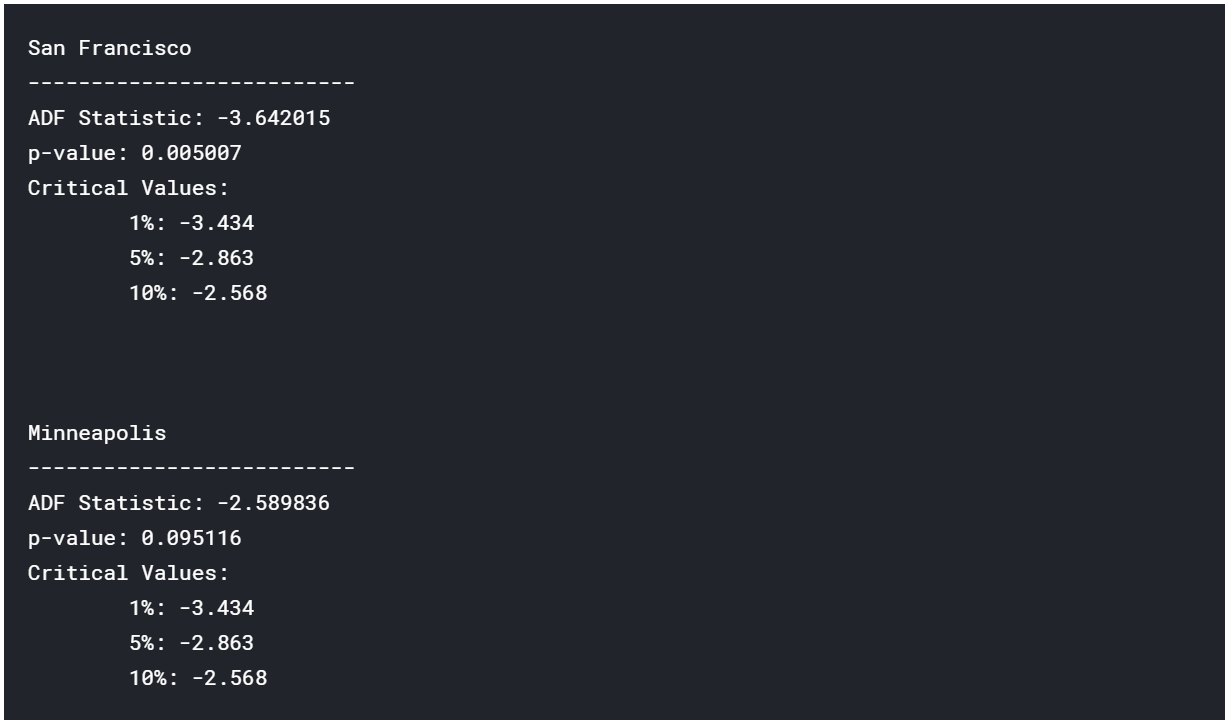So, what do any of these values mean?

• The ADF Statistic is the Augmented Dicken-Fuller score, the more negative this value is, the higher the certainty that we can reject out null hypothesis (the probability that the time series is stationary).
• p-value is the level of confidance for the null hypothesis. A usual threshold for this value is 0.05, meaning that if p_value <= 0.05 we can reject the null hypothesis.
• The rest of the values are the critical values for a 99%, 95% and 90% confidence intervals respectively.

So, what all this means in this case is that we can reject the null hypothesis for San Francisco since p is lower than 0.05, and also, the ADF score is lower than the limit for a 99% confidence interval. However, we fail to reject this hypothesis for Minneapolis, we could say it is stationarity with a confidence interval of 90%, but since the threshold was 95% (p = 0.05), we cannot reject it. This means we should difference the data before applying any model.

Some references to understand this tests can be found here:

### Autocorrelation

Last thing to check is if the data is autocorrelated. I want to use some autoregression methods to do forecasting in future posts, I can only do that if the data is autocorrelated (meaning that the value in a specific temporal point depends on previous values).

The statmodels library offers a great tool to check this. Everything outside of the shadowed area has a strong probability of being autocorrelated (over 95% confidence interval).

```import statsmodels.api as smprint('San Francisco')_=sm.graphics.tsa.plot_acf(data_mean['San Francisco'])plt.show()print('Minneapolis')_=sm.graphics.tsa.plot_acf(data_mean['Minneapolis'])plt.show()
```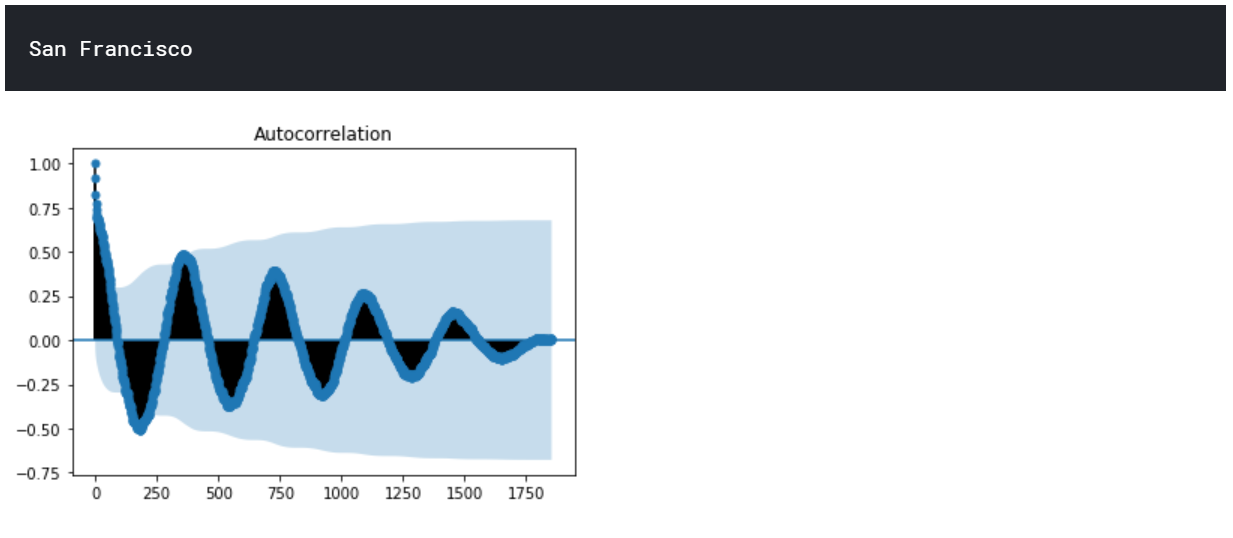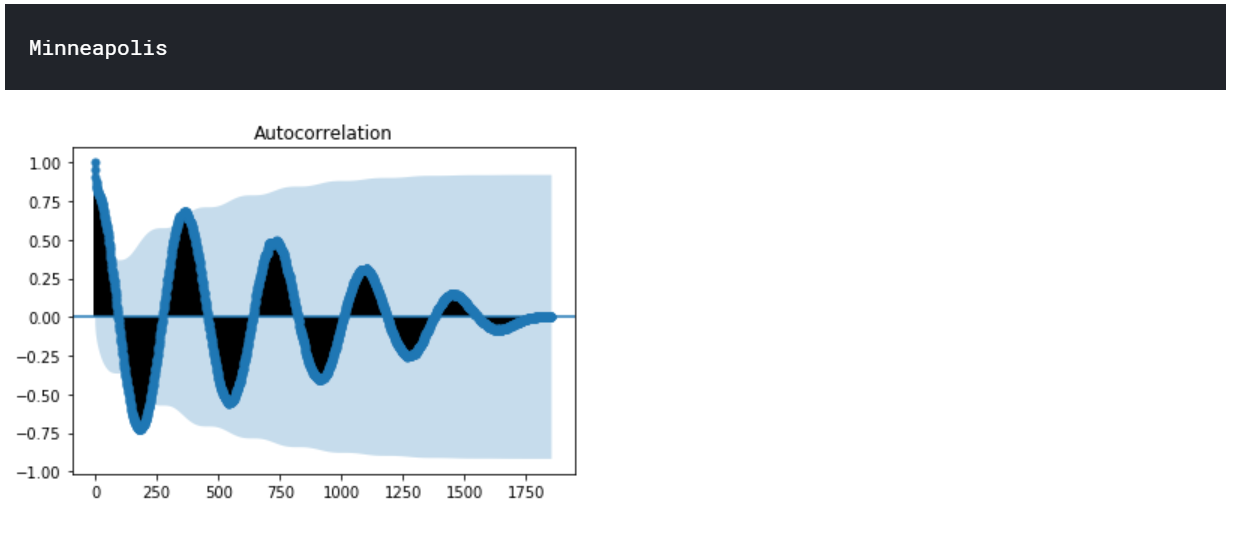Let’s focus on the most important lags (the ones closer to the point), for example, data from one-year range.

```import statsmodels.api as smprint('San Francisco')_=sm.graphics.tsa.plot_acf(data_mean['San Francisco'], lags=365)plt.show()print('Minneapolis')_=sm.graphics.tsa.plot_acf(data_mean['Minneapolis'], lags=365)plt.show()
```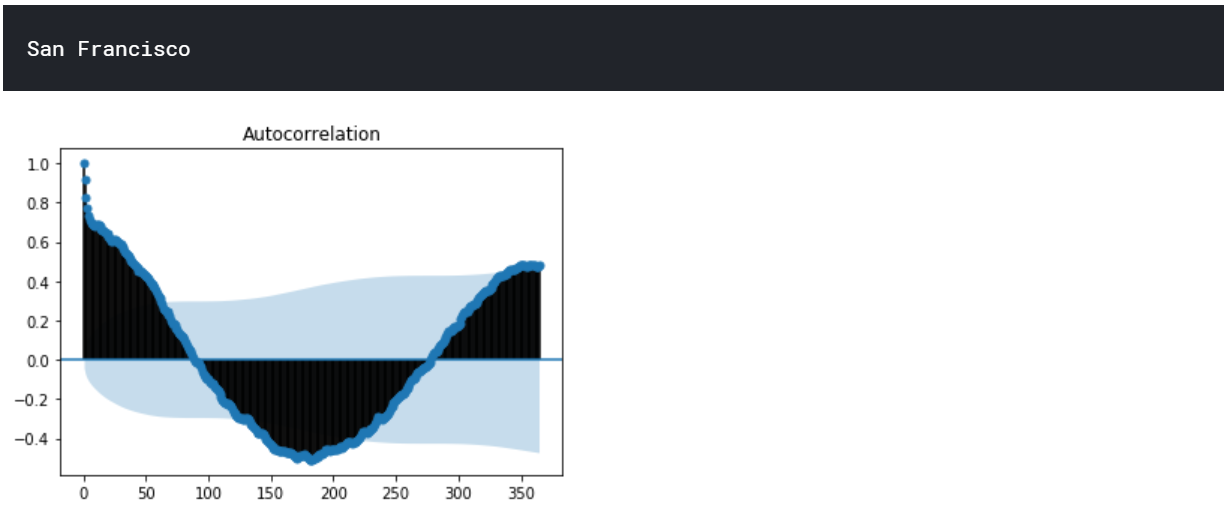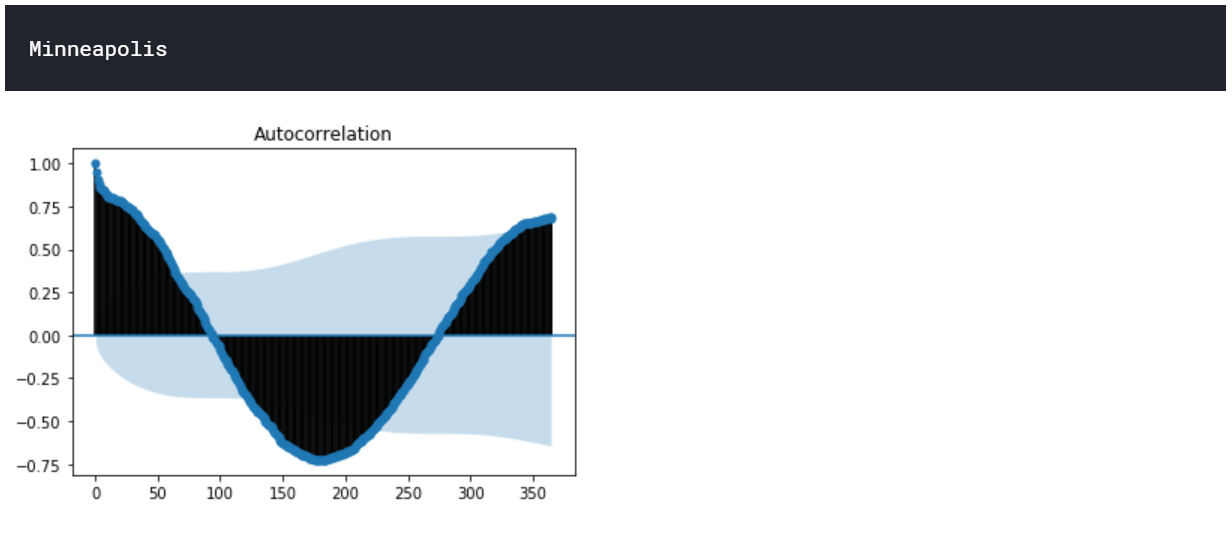We can also check for partial autocorrelation, which calculates the correlation removing the effect of any other previous point (points closer to the new value). Here further away points lose importance, I’ll focus on one-month range.

```print('San Francisco')_=sm.graphics.tsa.plot_pacf(data_mean['San Francisco'], lags=30)plt.show()print('Minneapolis')_=sm.graphics.tsa.plot_pacf(data_mean['Minneapolis'], lags=30)plt.show()
```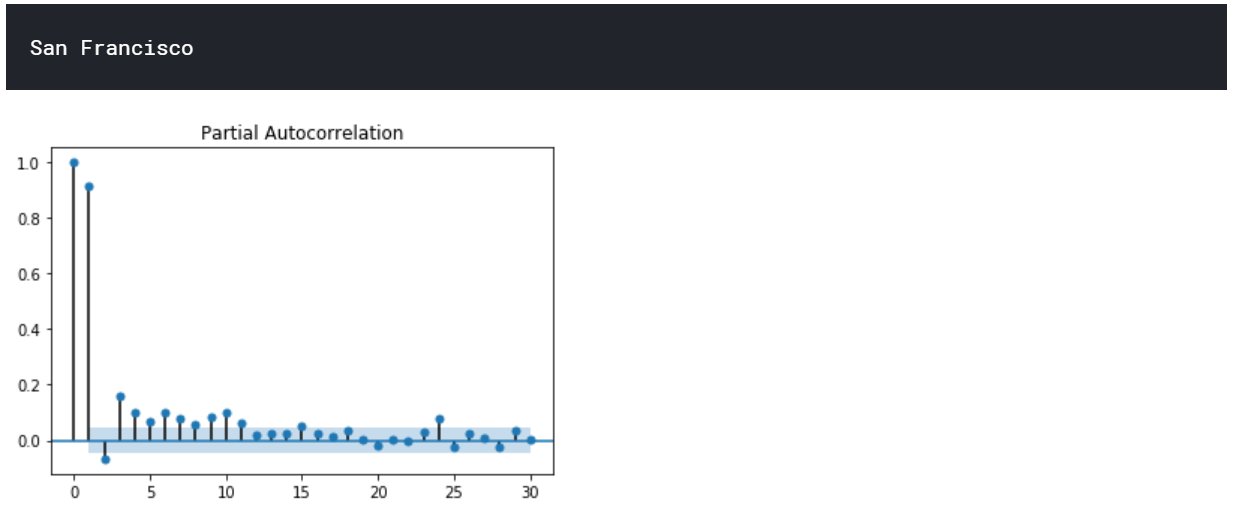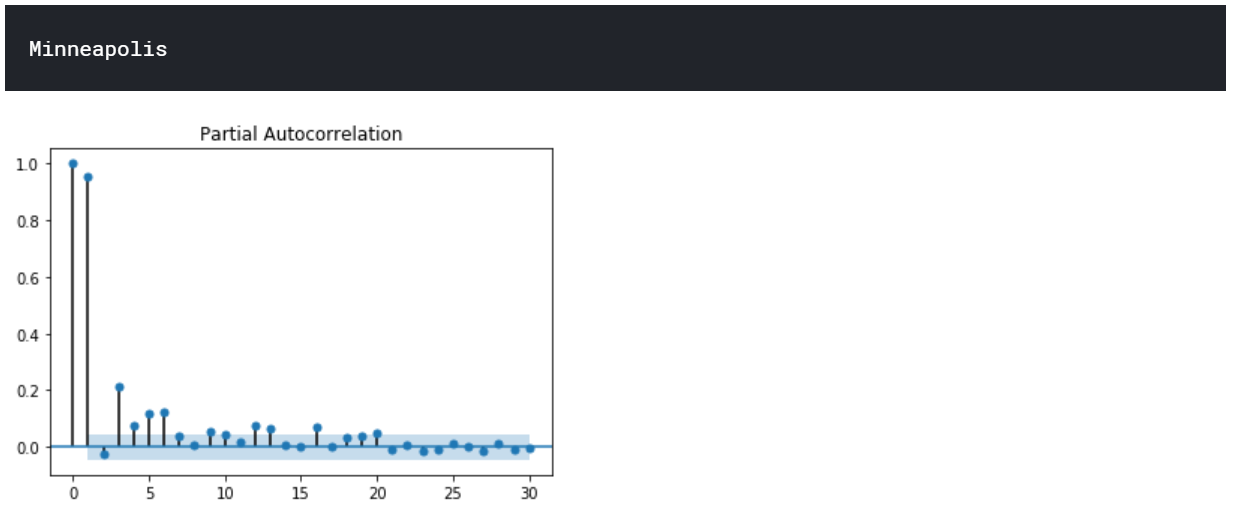Here are some references about autocorrelation:

### Trend — Seasonality decomposition

We can think about time series as a composition of trend, seasonality and residuals (noise or other random behaviour). The composition of the time series from this components can be bouth aditive or multiplicative:

• Additive: data = Trend + Seasonality + Residuals
• Multiplicative: data = Trend · Seasonality · Residuals

The Statsmodels package offers a function to extract this 3 component at once: Seasonal_decompose

The decomposition here is easy because we know the period to be 365 days.

```from statsmodels.tsa.seasonal import seasonal_decompose as sdsd_SF = sd(data_mean['San Francisco'], freq=365)sd_M = sd(data_mean['Minneapolis'], freq=365)
```
```_=plt.figure(figsize=(15,10))ax1=plt.subplot(311)_=ax1.plot(sd_SF.trend, label='San Francisco', alpha=0.7)_=ax1.plot(sd_M.trend, label='Minneapolis', alpha=0.7)_=plt.legend()ax2=plt.subplot(312)_=ax2.plot(sd_SF.seasonal, label='San Francisco', alpha=0.7)_=ax2.plot(sd_M.seasonal, label='Minneapolis', alpha=0.7)_=plt.legend()ax3=plt.subplot(313)_=ax3.plot(sd_SF.resid, label='San Francisco', alpha=0.7)_=ax3.plot(sd_M.resid, label='Minneapolis', alpha=0.7)_=plt.legend()
```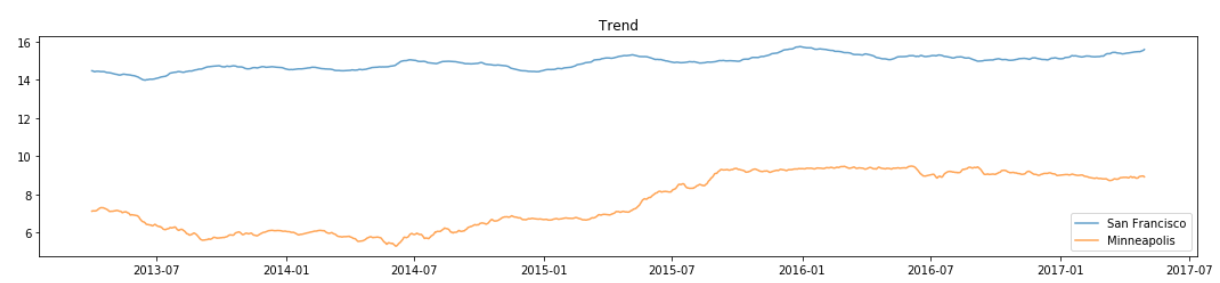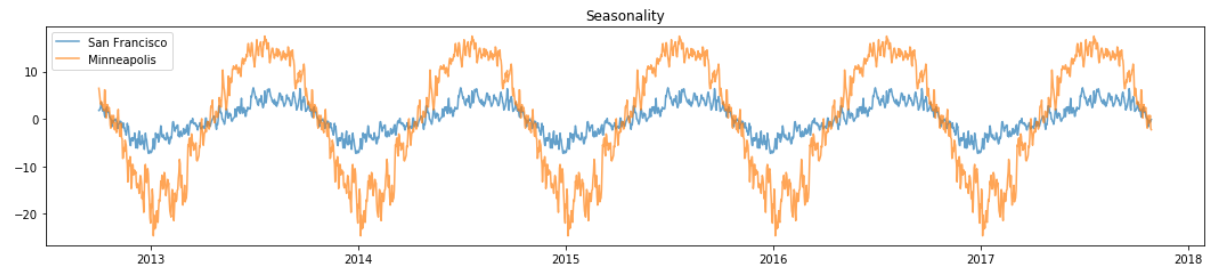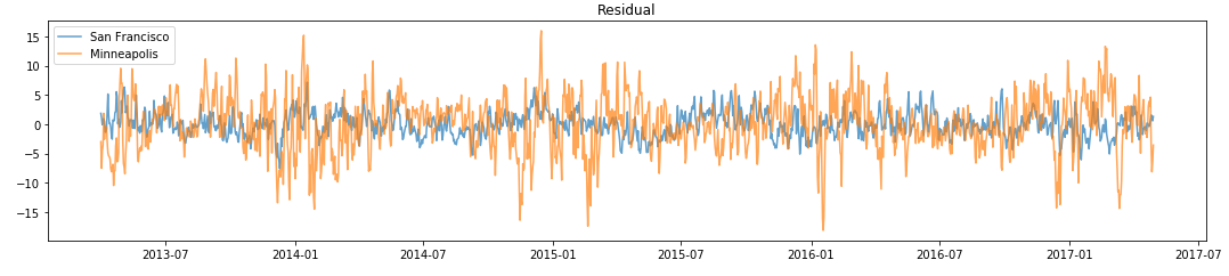Looking at this we can understand why we found the Minneapolis data to be non-stationary, there has been a clear increase in the temperature.

We can also find the trend by doing the moving average, I won’t do that here since it’s what the seasonal_decompose function does behing the scenes.

This is it for now. I’ll start with some forecasting algorithms in future posts.

I hope this can help some beginners like myself, thanks for reading! 🙂

Pd.: I would really appreciate some feedback